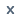Products

• KLQ6669

L × W × H : 6645×2270×2780(2950)

Seats : 23/22+1

• KLQ6668

L × W × H : 6645×2270×2780(2950)

Seats : 26/22+1, 23+1

• KLQ6702

L × W × H : 7020×2040×2645(2790)

Seats : 23/20+1, 21+1, 22+1, 18+1, 19+1

• KLQ6729

L × W × H : 7250×2270×2760(3000)

Seats : 28/25+1,26+1

• KLQ6759A

L × W × H : 7495×2270×2920(3030)

Seats : 30/26+1, 29+1

• KLQ6758A

L × W × H : 7545×2370×3020(3145)

Seats : 31/23+1, 26+1, 27+1, 28+1, 29+1

• KLQ6796

L × W × H : 7945×2450×3365

Seats : 35/29+1, 31+1+1

• KLQ6798

L × W × H : 7998×2450×3365

Seats : 35/29+1+1, 31+1+1

• KLQ6896

L × W × H : 8860×2450×3365

Seats : 39/35+1+1

• KLQ6898

L × W × H : 8949×2450×3365

Seats : 41/35+1+1, 37+1+1

• KLQ6920

L × W × H : 9225×2450×3365

Seats : 41/35+1+1/37+1+1